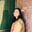Trusted answers to developer questions
Trusted Answers to Developer Questions

Related Tags

numpy
python

# What is numpy.positive() in Python?Umme Ammara

Grokking Modern System Design Interview for Engineers & Managers

Ace your System Design Interview and take your career to the next level. Learn to handle the design of applications like Netflix, Quora, Facebook, Uber, and many more in a 45-min interview. Learn the RESHADED framework for architecting web-scale applications by determining requirements, constraints, and assumptions before diving into a step-by-step design process.Python’s numpy.positive() method computes the positive of a number or array element-wise.

## Syntax

numpy.positive() is declared as shown below:

numpy.positive(x, /, out=None, *, where=True, casting='same_kind', order='K', dtype=None, subok=True[, signature, extobj]) = <ufunc 'positive'>


A universal function (ufunc) is a function that operates on ndarrays in an element-by-element fashion. The positive() method is a universal function.

## Parameters

The numpy.positive() method takes the following compulsory parameters:

• x [array-like﻿ or scalar﻿] - this is the input array.

The numpy.positive() method takes the following optional parameters:

 Parameter Description out Represents the location into which the output of the method is stored. If not provided or None, a freshly-allocated array is returned. where True value indicates that a universal function should be calculated at this position. casting Controls the type of datacasting that should occur. The same_kind option indicates that safe casting or casting within the same kind should take place. order Controls the memory layout order of the output function. The option K means reading the elements in the order they occur in memory. dtype Represents the desired data type of the array. subok Decides if subclasses should be made or not. If True, subclasses will be passed through.

## Return value

numpy.positive() returns the positive of the input, i.e. y = +x. The return type is an array or scaler depending on the input type.

## Examples

The examples below show the different ways numpy.positive() is used in Python.

### Positive of numbers

The code below outputs the numerical positive of -17.5 and 12. The result is shown below:

import numpy as npa = -17.5 b = -20print (np.positive(a))print (np.positive(b))

### Positive of arrays

The example below outputs the element-wise numerical positive of arrays arr1 and arr2:

import numpy as nparr1 = np.array([20,-30,40])arr2 = np.array([2,-3,4])print(np.positive(arr1))print(np.positive(arr2))

The example below outputs the element-wise numerical positive of arrays arr3 and arr4:

import numpy as nparr3 = np.array([[2.5,100,-10], [-2.9,90,89]])arr4 = np.array([[-2,-3,-4], [30,40,50]])print(np.positive(arr3))print(np.positive(arr4))

RELATED TAGS

numpy
python

CONTRIBUTORUmme Ammara